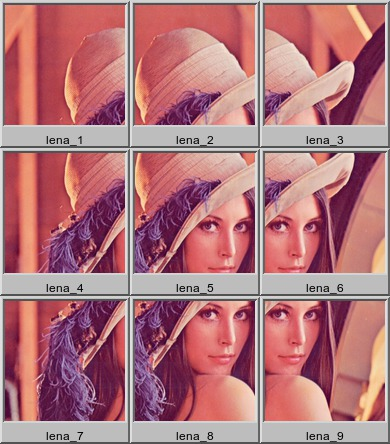## OVERLAPCROP

 Creates a sequence of cropped subsections permitting optional overlap of the subsections.

### EXAMPLES

 Matrix Mode Montage Original ImageArguments: -s 128 -o 50% -m matrix -M -L -RSequential Mode Montage -- Uniform Size Subsections Original ImageArguments: -s 120 -o 50% -m sequential -u -M -L -RSequential Mode Montage -- All Subsections Original ImageArguments: -s 120 -o 50% -m sequential -z 2 -M -L -RAnimation Mode -- Canvas Off Original ImageArguments: -s 120 -o 50% -m animation -d 50 -l 0 -c offAnimation Mode -- Canvas On Original ImageArguments: -s 120 -o 50% -m animation -d 50 -l 0 -c onWhat the script does is as follows for matrix output: Computes offsets and ending pixels for x and y Applies a loop for y and a loop for x, computing the subsection WxH+X+Y and crops the image, labeling appropriately This is equivalent to the following IM commands: outname=`echo "\$outfile" | sed -n 's/^\(.*\)[\.].*\$/\1/p'` suffix=`echo "\$outfile" | sed -n 's/^.*[\.]\(.*\)\$/\1/p'` ww=`identify -ping -format "%w" \$tmpA1` hh=`identify -ping -format "%h" \$tmpA1` sw=`echo "\${size}" | cut -dx -f1` sh=`echo "\${size}" | cut -dx -f2` overlap=`echo "\$overlap" | sed 's/%//g'` ox=`echo "\${overlap}" | cut -dx -f1` oy=`echo "\${overlap}" | cut -dx -f2` ox=`convert xc: -format "%[fx:floor(\$ox*\$sw/100)]" info:` oy=`convert xc: -format "%[fx:floor(\$oy*\$sh/100)]" info:` ow=`convert xc: -format "%[fx:\$sw-\$ox]" info:` oh=`convert xc: -format "%[fx:\$sh-\$oy]" info:` ew=`convert xc: -format "%[fx:\$ww-\$sw+\$ox-1]" info:` eh=`convert xc: -format "%[fx:\$hh-\$sh+\$oy-1]" info:` k=0 for ((i=0;i<=eh;i+=oh)); do for ((j=0;j<=ew;j+=ow)); do ((k++)) done done num=\$k t=1 k=\$initnum m=\$initnum dh=0 for ((i=0;i<=eh;i+=oh)); do dw=0 n=\$initnum for ((j=0;j<=ew;j+=ow)); do echo "Subsection: \$t out of \$num (\${sw}x\${sh}+\${dw}+\${dh}) row=\$m col=\$n" convert \$tmpA1[\${sw}x\${sh}+\${dw}+\${dh}] +repage \${outname}_r\${m}_c\${n}.\$suffix dw=\$((\$dw+\$ow)) ((t++)) ((k++)) ((n++)) done dh=\$((\$dh+\$oh)) ((m++)) done